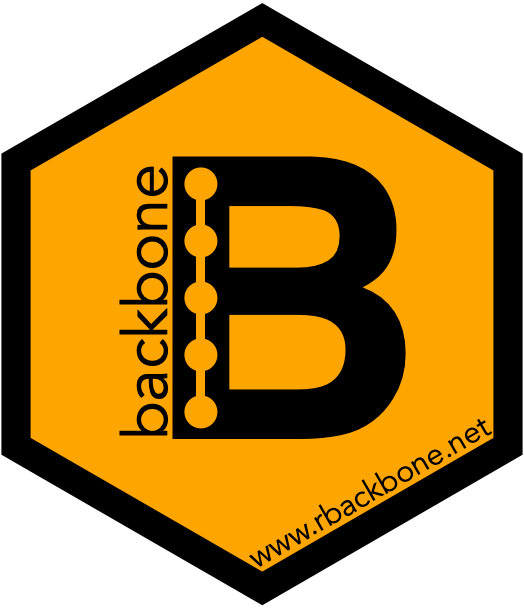# backbone## Welcome

Thank you for your interest in the backbone package! Here you will find a short example of how to use this package to extract the backbone of a bipartite projection. For more details on these functions and methods, please see our latest manuscripts on backbone here:

For additional resources on how to use the backbone package, please see www.rbackbone.net

## The Backbone of a Graph

The `backbone` package provides methods for extracting from a weighted graph a binary or signed backbone that retains only the significant edges. The user may input a weighted graph, or a bipartite graph from which a weighted graph is first constructed via projection. Backbone extraction methods include:

In a graph `G`, edges are either present (i.e. `G_{ij}=1`) or absent (i.e. `G_{ij}=0`). However in a weighted or valued graph, edges can take a range of values that may capture such properties as the strength or capacity of the edge. Although weighted graphs contain a large amount of information, there are some cases (e.g. visualization, application of statistical models not developed for weighted graphs) where it is useful to reduce this information by focusing on an unweighted subgraph that contains only the most important edges. We call this subgraph the backbone of `G`, which we denote as `G’`. Extracting `G’` from `G` requires deciding which edges to preserve. This usually involves selecting a threshold `T_{ij}` such that edges are preserved if they are above the threshold (i.e. `G_{ij}’=1` if `G_{ij} > T_{ij}`), and omitted if they are below the threshold (i.e. `G_{ij}’=0` if `G_{ij} < T_{ij}`). It is also possible to extract a signed backbone by selecting upper `T_{ij}` and lower `T’_{ij}` thresholds such that `G_{ij}’=1` if `G_{ij}>T_{ij}`, `G_{ij}’=-1` if `G_{ij} < T’_{ij}`, and `G_{ij}’=0` if `G_{ij} > T’_{ij}` and `G_{ij} < T_{ij}`. The key to all backbone extraction methods lies in the selection of `T`. The `backbone` package provides several different methods for selecting `T` and thus extracting `G’` from `G`.

The backbone package specifically provides five different ways of selecting `T` if the graph `G` is a bipartite projection. That is, `G = BB^T` where `B` is a bipartite graph. To decide whether an edge of a bipartite projection `G` is statistically significant, we compare the edge’s observed weight to the distribution of weights expected in a projection obtained from a random bipartite graph under a null model. Given a null model that can generate a distribution of an edge’s weights, we can then compute the probability that the observed weight is in the upper or lower tail of that distribution. The `backbone` package provides several different null models to use, all of which define different constraints on the row degrees and column degrees of a random bipartite graph in their corresponding distribution. The options are:

• `sdsm()`: computes the probability of edge weights being above or below the observed edge weights in a bipartite projection using the stochastic degree sequence model. Here the expected row and column degrees of a random bipartite graph in the distribution match that of the original bipartite graph.
• `fixedrow()`: computes the probability of edge weights being above or below the observed edge weights in a bipartite projection using the hypergeometric model. Here the row degrees of a random bipartite graph in the distribution exactly match that of the original bipartite graph. The column degrees are allowed to vary.
• `fixedcol()`: computes the probability of edge weights being above or below the observed edge weights in a bipartite projection using the Poisson binomial model. Here the column degrees of a random bipartite graph in the distribution exactly match that of the original bipartite graph. The row degrees are allowed to vary.
• `fdsm()`: computes the proportion of edge weights above or below the observed edge weights in a bipartite projection using the fixed degree sequence model. Here the row and column degrees of a random bipartite graph in the distribution exactly match that of the original bipartite graph.
• `fixedfill()`:computes the probability of edge weights being above or below the observed edge weights in a bipartite projection where the number of edges in a random bipartite graph of the distribution exactly match the number of edges of the original bipartite graph.

Once one of these models is computed, use to return the backbone graph for a chosen alpha value.

## Installation

You can install the released version of backbone from CRAN with:

``install.packages("backbone")``

You can install from GitHub with:

``````library(devtools)
install_github("domagal9/backbone", build_vignettes = TRUE)``````

## Example

This is a basic example which shows you how to solve a common problem using the included Davis’ Southern Women dataset. We can now decide which null model to compare the observed projection to.

Let’s compare our bipartite graph to the distribution of bipartite graphs where the expected row and column sums match our data. This is the `sdsm()` function.

``````library(backbone)
#>  ____
#> |  _ \   backbone v1.5.0
#> |#|_) |  Cite: Domagalski, R., Neal, Z. P., & Sagan, B. (2021). Backbone: An
#> |# _ <         R package for extracting the backbone of bipartite projections.
#> |#|_) |        PLoS ONE. https://doi.org/10.1371/journal.pone.0244363
#> |____/   For help: type vignette("backbone"); email zpneal@msu.edu; github domagal9/backbone
data(davis)
null_model_probabilities <- sdsm(davis)
#> This matrix object looks like an unweighted bipartite network of 18 agents and 14 artifacts.
backbone <- backbone.extract(null_model_probabilities, signed = TRUE, alpha = 0.05)``````

For more detailed examples and background on the topic, see `vignette("backbone_introduction", package = "backbone")` or our manuscript on the backbone package: https://doi.org/10.1371/journal.pone.0244363